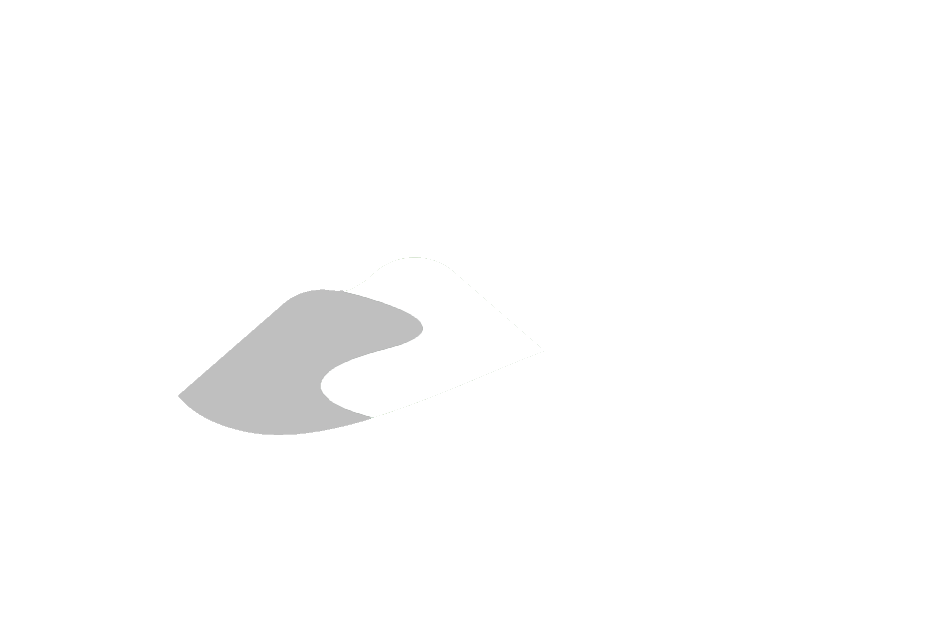View on GitHub

# General Information

## Oak Ridges Moraine Groundwater Program

Shallow water overland flow

Lateral movement of water over the land surface

# Lateral movement of land surface moisture

The simulation of lateral water movement is approximated by the local inertial approximation (LIA) of the shallow water equations (SWE) of de Almeda et.al., 2012; 2013.

It is important to note that the interpretation of the use of the LIA to the SWE does not include the physical movement of sheet flow, rather, the LIA is being interpreted as a means of moving water laterally, either thought a network of micropores and rill structures. That being said, roughness coefficients may be higher than typically associated with flood forecasting. only when water depths achieve appreciable depths (say greater than 1cm) will the interpretation of the LIA as representing depth-averaged shallow surface water movement hold. Under most conditions, however, flow depths, on average, will report depths much less than 1cm. As flow depths receded beyond this (albeit arbitrary) threshold, flow paths through macropore networks and rill structures are expected to lengthen relative to the grid cell dimension and is assumed to be subcritical. Fortunately, the solution of de Almeda et.al., (2012) is best applicable to low Froude numbers, $Fr<0.5$, thus suitable for the interpretation made here.

## Governing Equations

The shallow water (i.e., depth-averaged) approximation of incompressible fluid flow in two-dimension can be solved using the following system of equations (de Almeda et.al., 2013):

### Conservation of mass

$\frac{\partial h}{\partial t} + \frac{\partial q_x}{\partial x} + \frac{\partial q_y}{\partial y} = 0$

### Conservation of momentum

$\underbrace{\frac{\partial q_x}{\partial t}}_{\substack{\text{local} \\\text{acceleration}}} + \underbrace{\frac{\partial}{\partial x}\left(uq_x\right) + \frac{\partial}{\partial y}\left(vq_x\right)}_{\substack{\text{convective} \\\text{acceleration}}} + \underbrace{gh\frac{\partial \left(h+z\right)}{\partial x}}_{\substack{\text{pressure +} \\\text{bed gradients}}} + \underbrace{ghS_{f_x}}_{\text{friction}} = 0$ $\underbrace{\frac{\partial q_y}{\partial t}}_{\substack{\text{local} \\\text{acceleration}}} + \underbrace{\frac{\partial}{\partial y}\left(vq_y\right) + \frac{\partial}{\partial x}\left(uq_y\right)}_{\substack{\text{convective} \\\text{acceleration}}} + \underbrace{gh\frac{\partial \left(h+z\right)}{\partial y}}_{\substack{\text{pressure +} \\\text{bed gradients}}} + \underbrace{ghS_{f_y}}_{\text{friction}} = 0$

in de Almeda et.al., (2012), friction slope ($S_f$) is approximated using the Manning-Strickler equation and assuming wide shallow cross-sectional area normal flow (i.e., $R\approx h$), where:

$\sqrt{S_f} = \frac{nq}{h^{5/3}}$

thus yielding:

$\frac{\partial q_x}{\partial t} + \frac{\partial}{\partial x}\left(uq_x\right) + \frac{\partial}{\partial y}\left(vq_x\right) + gh\frac{\partial \left(h+z\right)}{\partial x} + \frac{gn^2\Vert \mathbf{q}\Vert q_x}{h^{7/3}} = 0$ $\frac{\partial q_y}{\partial t} + \frac{\partial}{\partial y}\left(vq_y\right) + \frac{\partial}{\partial x}\left(uq_y\right) + gh\frac{\partial \left(h+z\right)}{\partial y} + \frac{gn^2\Vert \mathbf{q}\Vert q_y}{h^{7/3}} = 0$

## Numerical Scheme

$q^{n+1}_{i-1/2} = \frac{\theta q^n_{i-1/2}+\frac{1-\theta}{2}\left(q^n_{i-3/2}+q^n_{i+1/2}\right) - gh^n_f \frac{\Delta t}{\Delta x}\left(\eta^n_i - \eta^n_{i-1}\right)} {1 + g \Delta t n^2 ||\vec{q}^n_{i-1/2}||/h^{7/3}_{f}}$

where

$||\vec{q}^n_{i-1/2}|| = \sqrt{\left(q^n_{x,i-1/2}\right)^2 + \left(q^n_{y,i-1/2}\right)^2},$

Courant-Friedrichs-Lewy condition:

$\Delta t = \alpha \frac{\Delta x}{\sqrt{gh_\text{max}}}, \qquad 0<\alpha\leq 1$

after updating fluxes for time step $n+1$, cell heads are updated by:

$\eta^{n+1}_{i,j} = \eta^n_{i,j} + \frac{\Delta t}{\Delta x}\left(q_x|^{n+1}_{i-1/2,j}-q_x|^{n+1}_{i+1/2,j}+q_y|^{n+1}_{i,j-1/2}-q_y|^{n+1}_{i,j+1/2}\right)$

hosted on Github